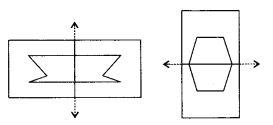## MP Board Class 6th Maths Solutions Chapter 13 Symmetry Ex 13.2

Question 1.
Find the number of lines of symmetry for each of the following shapes: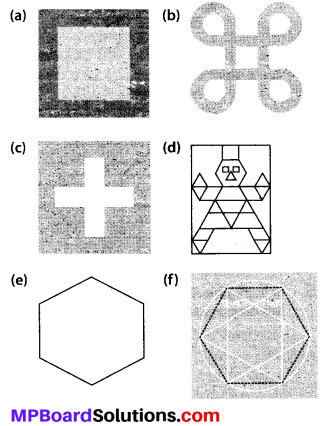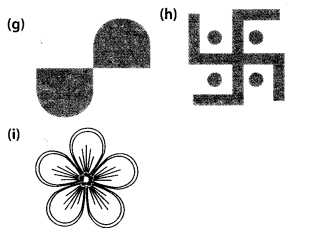Solution:
(a)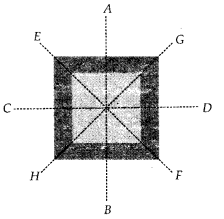Lines of symmetry are AB, CD, EF and GH.
∴ Number of lines of symmetry = 4

(b)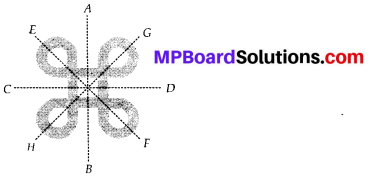Lines of symmetry are AB, CD, EF and GH.
∴ Number of lines of symmetry = 4

(c)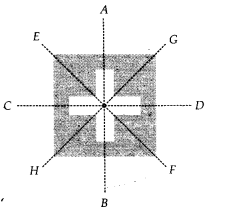Lines of symmetry are AB, CD, EF and GH.
∴ Number of lines of symmetry = 4

(d)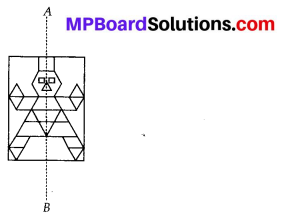Lines of symmetry is AB.
∴ Number of lines of symmetry = 1

(e)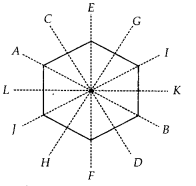Lines of symmetry are AB, CD, EF, GH, IJ and KL.
∴ Number of lines of symmetry = 6

(f)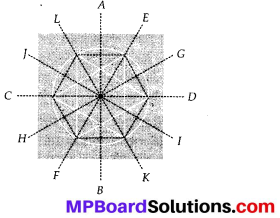Lines of symmetry are AB, CD, EF, GH, IJ and KL.
∴ Number of lines of symmetry = 6
(g) No lines of symmetry.
(h) No lines of symmetry.
(i)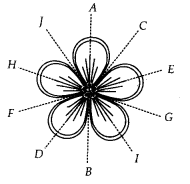Lines of symmetry are AB, CD, EF, GH and IJ.
∴ Number of lines of symmetry = 5

Question 2.
Copy the triangle in each of the following figures on squared paper. In each case, draw the line(s) of symmetry, if any and identify the type of triangle. (Some of you may like to trace the figures and try paper-folding first!)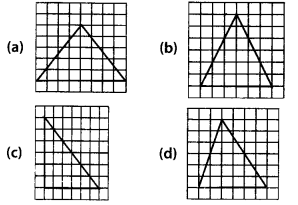Solution: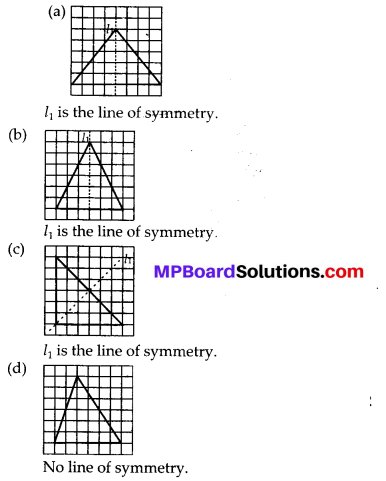Question 3.
Complete the following table.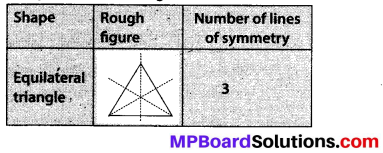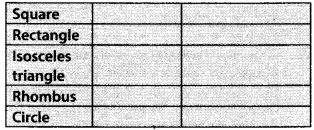Solution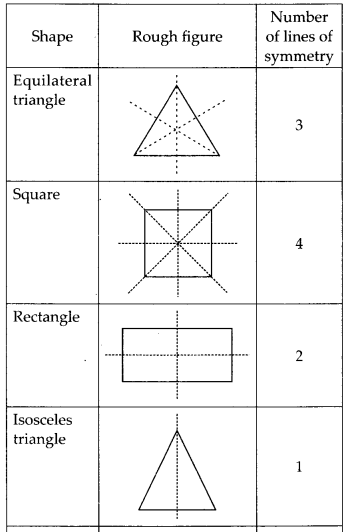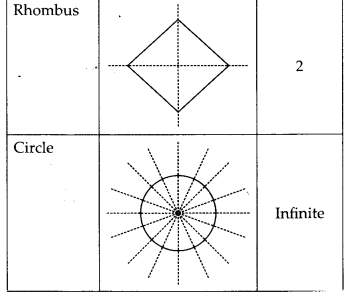Question 4.
Can you draw a triangle which has
(a) exactly one line of symmetry?
(b) exactly two lines of symmetry?
(c) exactly three lines of symmetry?
(d) no lines of symmetry?
Sketch a rough figure in each case.
Solution:
(a) Yes, an isosceles triangle has one line of symmetry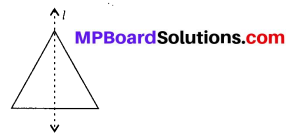(b) No, triangle cannot be formed which has exactly two lines of symmetry.
(c) Yes, equilateral triangle has three lines of symmetry.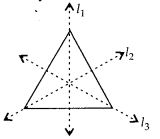(d) Yes, scalene triangle has no lines of symmetry.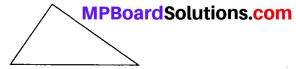Question 5.
On a squared paper, sketch the following:
(a) A triangle with a horizontal line of symmetry but no vertical line of symmetry.
(b) A quadrilateral with both horizontal and vertical lines of symmetry.
(c) A quadrilateral with a horizontal line of symmetry but no vertical line of symmetry.
(d) A hexagon with exactly two lines of symmetry.
(e) A hexagon with six lines of symmetry.
(Hint: It will be helpful if you first draw the lines of symmetry and then complete the figures.)
Solution: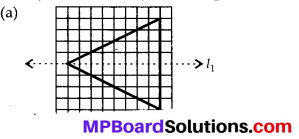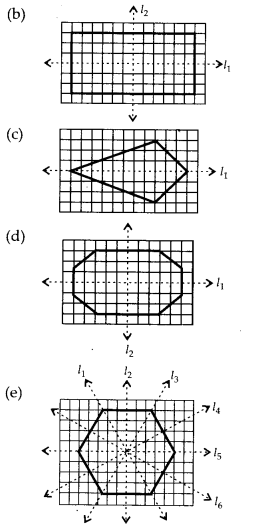Question 6.
Trace each figure and draw the lines of symmetry, if any: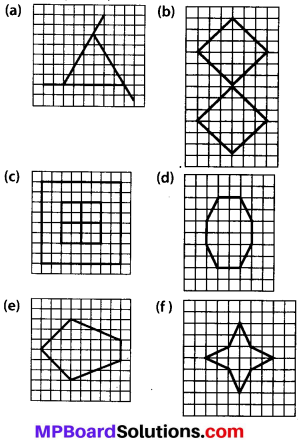Solution: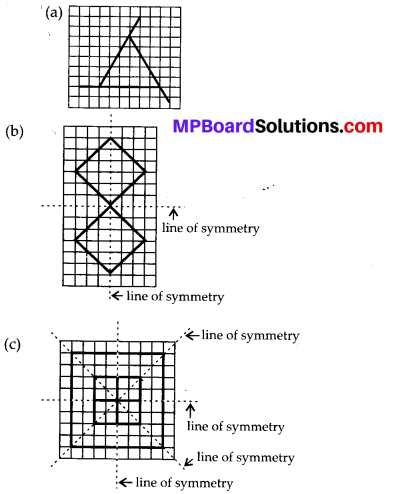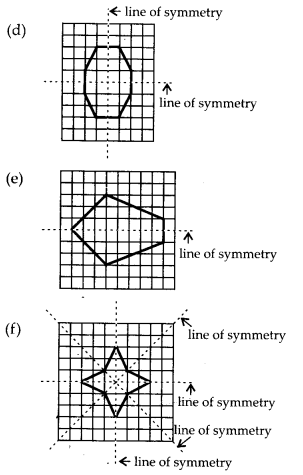Question7.
Consider the letters of English alphabets, A to Z. List among them the letters which have
(a) vertical lines of symmetry (like A)
(b) horizontal lines of symmetry (like B)
(c) no lines of symmetry (like Q)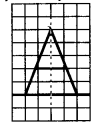Solution:
(a) The letters which have vertical lines of symmetry are A, H, I, M, O, T, U, V, W, X, Y.
(b) The letters which have horizontal lines of symmetry are B, C, D, E, H, I, K, O, X.
(c) The letters which have no line of symmetry are F, G, J, L, N, P, Q, R, S, Z

Question 8.
Given here are figures of a few folded sheets and designs drawn about the fold. In each case, draw a rough diagram of the complete figure that would be seen when the design is cut off.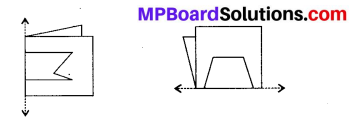Solution: